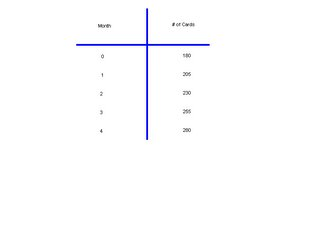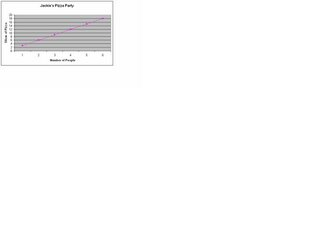## Monday, May 22, 2006

### My Growing Post

1.(a) Jack will have the total of 280 cards at the end of the next 4 months.(b) 180+25(C)= #of cards 180+25(4)=280 cards
First I started off by multiplying the number of months, and is 4 by 25 the number of cards he buys after each month. Then I added his remaing 180 cards to get the total. Then he will have 280 cards at the end of the next 4 months.

(c) Jack will have the total of 1380 cards when he turns 12.
I got the answer by finding out the difference between 8 and 12, and it's 4. I multiplied 4 by 12 and I got 48 because there are 48 months in 4 years. Then I used the algebraic formula, 180+25(48). All that I did was change the variable. Then I multiplied 48 by 25 to give me 1200, and then I added the remaining 180 to get 1380 cards.

2.(a) Jackie should order the total of 2 pizzas. She should order 2 pizzas because if you look at the chart and find 4 people there will be 12 slices and if there are 6 slices in each pizza I divide 12 by 6 and that gave me 2 whole pizzas.(b) If two more people show up at the party Jackie should order 1 more pizza making the total of 3 pizzas. She should order 3 pizzas in total becuase if you look at the chart and find 6 people there will be 18 slices. I divided 18 slices by 6 to get the total of 3 pizzas

3.(a) Cathy will have \$200.00 dollars in her saving account. I got the answer by taking the formula of 20+15(12). I mulitplied 12 by 15 to get 180 then I added her \$20.00 dollars to get \$200.00 dollars.

(b) If Cathy made a mistake and she had actually had \$25.00 dollars instead of \$20.00 the formula would be 25+15C= savings account. Then Cathy would have a total of \$205.00 dollars for that year.

4.(a) 3n+4n+7=

2n+123(1)+4(1)+7=

2(1)+123+4+7=14

N= 1

b) 8n-(4+9)=11
8(3)-(4+9)=11
24-13=11
N= 3

(c) (8-3)n+7n+8=

4n+405n+7n+8=

4n+405(4)+7(4)+8=

4(4)+4020+28+8=56

N= 4

At 5/22/2006 10:51 AM,jay said...

good job candace!!=)

At 5/22/2006 12:14 PM,amelia_ mal said...

hey Candac! That was great u have given me help. it's Amalia.

At 5/22/2006 6:59 PM,Candy said...

This comment has been removed by a blog administrator.

At 5/24/2006 11:40 AM,kimbutt said...

Hey Candace.You did a good job.

At 5/24/2006 12:06 PM,Mr. Reece said...

Great job with the first three questions Candy. Remember that question 4 is on the this week's growing post

At 6/19/2006 3:09 PM,Mr. Reece said...